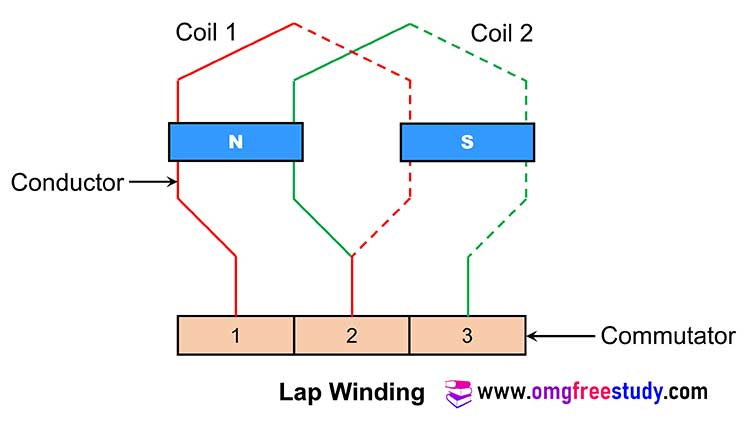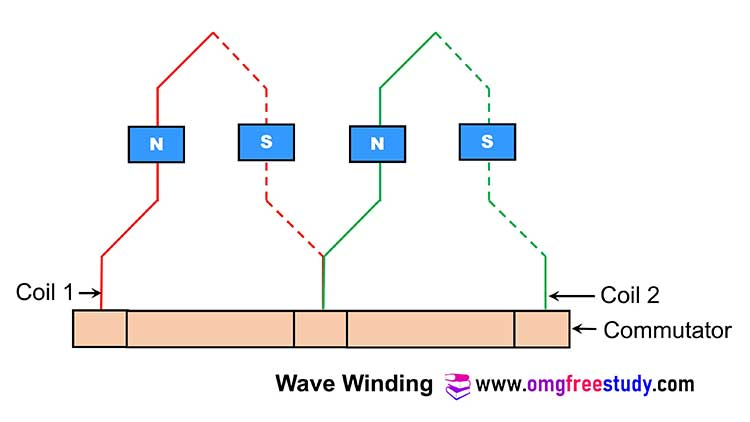# Types of Armature Winding | Lap Winding and Wave Winding

In dc machine there are two windings present that is armature winding and field winding. And armature winding is basically divided into two type as per connection of winding conductor.

## Types of Armature Windings

• Depending on the way of connecting the conductors, the armature winding is classified into two types that is lap winding and wave winding.

### Lap armature Winding

• The basic connection of lap winding is shown in fig.
• For lap winding the number of parallel paths is exactly equal to the number of poles P.
• Number of parallel patha(A) = Numberof Poles(P)• The lap winding mostly is useful for low voltage and high current machines.
• In this type of winding large number of parallel paths that means the lap wound armature winding is capable of supplying larger load currents.
• Hence the generators of high current capacity use the lap wound armature winding. But these generators are generated low capacity voltage.
• In lap winding the armature conductors are divided into P groups (number of poles). All the conductors in a group are connected in series and all such groups are connected in parallel.

### Wave Armature Winding

• The basic connection of wave winding is shown in fig.• This type of winding will create only two parallel paths independent of the number of poles.
• Number of parallel path (A) = 2
• Wave winding is useful for high voltage low current capacity machines
• Note that the number of parallel paths for wave winding will be less than that for lap winding.
• Hence this winding does not have the capability of supplying larger load currents. So the generators with wave winding are low current ratings and these generators are capable of producing high voltage.
• Thus in wave winding, all the conductors are connected in series to form a single closed circuit.

## Comparison of Lap and Wave Windings:

Que. Compare lap winding and wave winding on the basis of : i) Number of parallel paths in the winding. ii) voltage generating capability  iii) Current sourcing capability iv) Number of brush sets.

 Parameters Lap winding Wave winding Number of parallel paths in the winding. A =P (A= No. of parallel path & P= No. of poles) A=2 always  (A= No. of parallel path) voltage generating capability Low High Current sourcing capability High Low Number of brush sets. Equal to number of pole Equal to 2 always

Watch MCQ Question Lecture on DC Motor and DC Gen. on YouTube by clicking here.

Scroll to Top
Scroll to Top